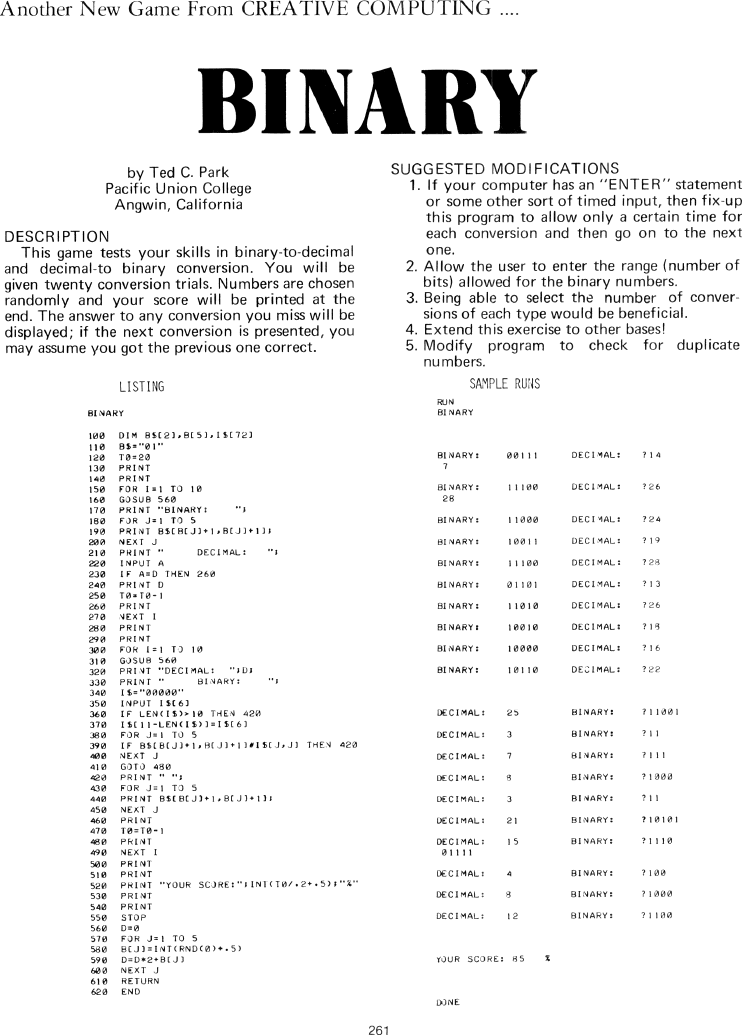The Best of Creative Computing Volume 1 (published 1976)

 Page 261 << PREVIOUS >> NEXT Jump to page: Go to contents Go to thumbnails

Binary (BASIC computer game, binary to decimal conversion, decimal to binary conversion, BASIC program listing, sample run)Another New Game From CREATIVE COMPUTING

BINARY

by Ted C. Park
Pacific Union College
Angwin, California

DESCRIPTION
This game tests your skills in binary-to-decimal and decimal-to binary
conversion. You will be given twenty conversion trials. Numbers are chosen
randomly and your score will be printed at the end. The answer to any conversion
you miss will be displayed; if the next conversion is presented, you
may assume you got the previous one correct.

LISTING

BINARY

100 DIM B\$(2),B(5),I\$(72)
110 B\$="01"

120 T0=20
130 PRINT
140 PRINT
150 FOR I=1 TO 10
160 GOSUB 560
170 PRINT "BINARY:     ";
180 FOR J=1 TO 5
190 PRINT B\$(B(J)+1,B(J)+1);
200 NEXT J
210 PRINT "     DECIMAL:     ";
220 INPUT A
230 IF A=D THEN 260
240 PRINT D
250 TO=TO-1
260 PRINT
270 NEXT I
280 PRINT
290 PRINT
300 FOR I=1 TO 10
310 GOSUB 560
320 PRINT "DECIMAL:   ";D;
330 PRINT "    BINARY:    ";
340 I\$="00000"

350 INPUT I\$(6)
360 IF LEN(I\$)>10 THEN 420
370 I\$(11-LEN(I\$))=I\$(6)
380 FOR J=1 TO 5
390 IF B\$(B(J)+1,B(J)+1)#1\$(J,J) THEN 420
400 NEXT J
410 GOTO 480
420 PRINT " ";
430 FOR J=1 TO 5
440 PRINT B\$(B(J)+1,B(J)+1);
450 NEXT J
460 PRINT
470 TO=TO-1
480 PRINT
490 NEXT I
500 PRINT
510 PRINT

530 PRINT
540 PRINT
550 STOP
560 D=0
570 FOR J=1 TO 5
580 B(J)=INT(RND(0)+.5)
590 D=D*2+B(J)
600 NEXT J
610 RETURN
620 END

SUGGESTED MODIFICATIONS

1. If your computer has an "ENTER" statement or some other sort of timed input,
then fix-up this program to allow only a certain time for each conversion and
then go on to the next one.

2. Allow the user to enter the range (number of bits) allowed for the binary
numbers.

3. Being able to select the number of conversions of each type would be
beneficial.

4. Extend this exercise to other bases!

5. Modify program to check for duplicate numbers.

SAMPLE RUNS

RUN
BINARY

BINARY: 00111 DECIMAL: ?14
7

BINARY: 11100 DECIMAL: ?26
28

BINARY: 11000 DECIMAL: ?24

BINARY: 10011 DECIMAL: ?19

BINARY: 11100 DECIMAL: ?28

BINARY: 01101 DECIMAL: ?13

BINARY: 11010 DECIMAL: ?26

BINARY: 10010 DECIMAL: ?18

BINARY: 10000 DECIMAL: ?16

BINARY: 10110 DECIMAL: ?22

DECIMAL: 25 BINARY: ?11001

DECIMAL: 3 BINARY: ?11

DECIMAL: 7 BINARY: ?111

DECIMAL: 8 BINARY: ?1000

DECIMAL: 3 BINARY: ?11

DECIMAL: 21 BINARY: ?10101

DECIMAL: 15 BINARY: ?1110
01111

DECIMAL: 4 BINARY: ?100

DECIMAL: 8 BINARY: ?1000

DECIMAL: 12 BINARY: ?1100

DONE

 Page 261 << PREVIOUS >> NEXT Jump to page: Go to contents Go to thumbnails